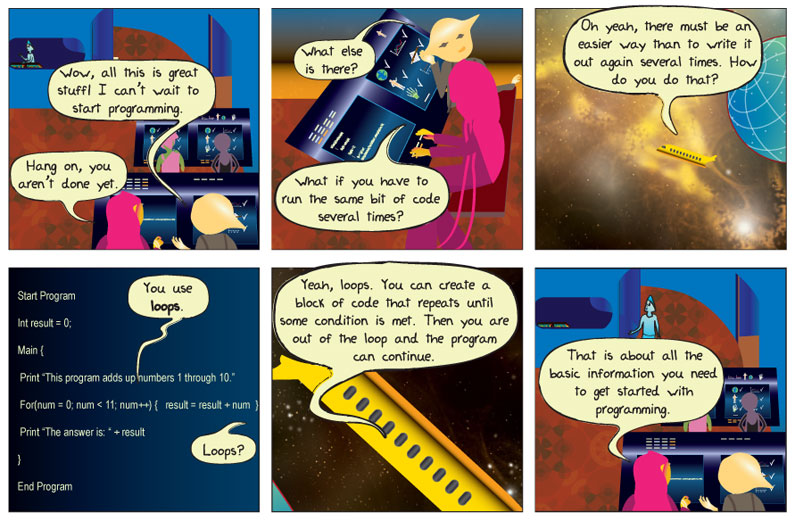## Eposode 151: Loops## Comic Transcript

Panel 1.
Ludd: Wow, all this is great stuff! I can’t wait to start programming.
Kodu: Hang on, you aren’t done yet.

Panel 2.
Ludd: What else is there?
Kodu: What if you have to run the same bit of code several times?

Panel 3.
Ludd: Oh yeah, there must be an easier way than to write it out again several times. How do you do that?

Panel 4.
Kodu: You use loops.
Ludd: Loops?

Panel 5.
Kodu: Yeah, loops. You can create a block of code that repeats until some condition is met. Then you are out of the loop and the program can continue.

Panel 6.
Kodu: That is about all the basic information you need to get started with programming.

## What does it mean?

Loop – is a block of computer code that can repeat for a fixed number of times, or until some condition is met, or forever.

The ability for a computer program to repeat a section of code is an essential part of any computer programming language. There are several reasons for repeating code; one example might require a block of code to update a variable that changes a certain amount each time. An example might be the angle at which a projectile is launched at a fixed speed such that the projectile travels a maximum distance.

Another example for using loops is in remotely controlled robots. In order for the robot to be able to receive input from the user, it needs to continuously check to see if the user has pushed a button or a joystick that causes the robot do something. Therefore, there must be a block of code that runs continuously in a loop looking for that input.

Let’s look at an example of a loop in a program.

Start Program

Int result = 0;

Main {

Print “This program adds up numbers 1 through 10.”

For(num = 0; num < 11; num++) {           result = result + num      }      Print “The answer is: “ + result } End Program

The following is an example of a “For” programming loop.

For(num = 0; num < 11; num++) {

This line of code translates into “For the variable ‘num’ starting with 0 (num = 0), while it is less than 11 (num < 11), increase its value by 1 (num++).” The loop will be broken as soon as the value of “num” becomes greater than 10. Because this is a very common type of code that programmers use, it has been simplified into one line to make programming easier.

result = result + num

This line simply means for the program to take the current value of the variable “result” (appearing on the right side of the statement), add the value of “num” to it and assign the outcome to the variable “result,” which can be used next time the loop executes. It should be noted that the equal sign (=) in this instance is an assignment operator and should not be confused with a mathematical equation. If it were, then this statement would only be true if “num” was equal to 0.